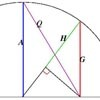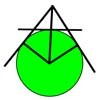# Search by Topic

#### Resources tagged with Arithmetic, Geometric and Harmonic Means similar to Classical Means:

Filter by: Content type:
Age range:
Challenge level:

### There are 7 results

Broad Topics > Algebraic expressions, equations and formulae > Arithmetic, Geometric and Harmonic Means### Classical Means

##### Age 16 to 18 Challenge Level:

Use the diagram to investigate the classical Pythagorean means.### Without Calculus

##### Age 16 to 18 Challenge Level:

Given that u>0 and v>0 find the smallest possible value of 1/u + 1/v given that u + v = 5 by different methods.### AMGM

##### Age 14 to 16 Challenge Level:

Can you use the diagram to prove the AM-GM inequality?### Three Ways

##### Age 16 to 18 Challenge Level:

If x + y = -1 find the largest value of xy by coordinate geometry, by calculus and by algebra.### Pythagorean Golden Means

##### Age 16 to 18 Challenge Level:

Show that the arithmetic mean, geometric mean and harmonic mean of a and b can be the lengths of the sides of a right-angles triangle if and only if a = bx^3, where x is the Golden Ratio.### Mean Geometrically

##### Age 16 to 18 Challenge Level:

A and B are two points on a circle centre O. Tangents at A and B cut at C. CO cuts the circle at D. What is the relationship between areas of ADBO, ABO and ACBO?### About Pythagorean Golden Means

##### Age 16 to 18

What is the relationship between the arithmetic, geometric and harmonic means of two numbers, the sides of a right angled triangle and the Golden Ratio?